# Seminar 8

In this seminar, we will use Y.RData and PRC.RData files that we created in the Seminar 2. We will focus on simulating VaR for options using Monte Carlo method.

The plan for this week:

The following section is material for students to learn by themselves: Calculating VaR for a portfolio of stock and option

## Using Black-Scholes to price an option¶

We will define a bs() function that takes a strike price, $K$, the price of the underlying asset $P$, the annual risk-free interest rate, $r$, annual volatiliry $\sigma$, and the time to maturity. This function can return the price of a European put or call option (put by default) will return the price of a European put option. We will focus only on put options in this section, but the process is analogous for call options:

In [ ]:
# Black-Scholes function(default type of option is put option)
bs <- function(K, P, r, sigma, Maturity, type = "Put"){
d1 <- (log(P/K) + (r+0.5*sigma^2)*(Maturity))/(sigma*sqrt(Maturity))
d2 <- d1 - sigma*sqrt(Maturity)
Call <- P*pnorm(d1) - K*exp(-r*(Maturity))*pnorm(d2)
Put <- K*exp(-r*(Maturity))*pnorm(-d2) - P*pnorm(-d1)
if (type == "Put") {
return(Put)
} else if (type == "Call") {
return(Call)
} else {
return("Not a valid type")
}
}


We will work with the JP Morgan stock. Let's first load it into our environment and estimate its volatility:

In [ ]:
# Load JPM stock
y <- Y$JPM plot(y, type = "l", main = "JPM stock")  We need to scale the standard deviation of the stock by$\sqrt{250}$to get the estimated yearly volatility. We use 250 because it is the number of trading days in a year (Monday - Friday). Note that this is different from the calendar days, which are 365. The latter are used when dealing with the yearly risk-free interest rate. In [ ]: # Estimating volatility with returns n <- length(y) sigma <- sd(y)*sqrt(250) sigma  In order to use the Black-Scholes formula, we need the underlying price of the asset. We will load the PRC.RData file and get the last observation available for JP Morgan: In [ ]: # Getting the last price available for JPM load("PRC.RData") P <- tail(PRC$JPM,1)
P


We need to make assumptions on the risk-free rate and necessary parameters for an option. We will assume the risk-free rate is $r=3\%$, the maturity is 1 year, and the strike price is $K=100$:

In [ ]:
# Assuming the necessary parameters
r <- 0.03
Maturity <- 1
K <- 100


Now we can use the bs() function we defined to price the option:

In :
# Calculating the option price
put <- bs(K = K, P = P, r = r, sigma = sigma, Maturity = Maturity)
put

4.11663091718259

#### Option price as a function of the Underlying stock price¶

We have used the last price observed to calculate the price of the option.

However, we can do a plot of the option price as a function of the underlying stock price. To do so, we will create a variable called x that will contain a sequence of values between 50 and 150. You can think of this variable as the x-axis of the plot. These are all the possible underlying prices we want will consider. We will use the function seq() with a length of 1000. Then, we will call the option bs() with the variable x as the argument P, for underlying price, and the result will be a vector of length 1000, with the option price for each value in the sequence x. This result is the y-axis of the plot. Finally, we plot them together:

In :
# Option price as a function of underlying stock price

# Sequence of underlying prices
x <- seq(50, 150, length = 1000)

# Plot option price
plot(x, bs(K = K, P = x, r = r, sigma = sigma, Maturity = Maturity),
type = "l", ylab = "Option", xlab = "Underlying price", lwd = 3, col = "pink", las = 1,
bty = "l", main = "Price of put option depending on underlying stock price")

# Add a point for the observed price
points(P, bs(K=K, P=P, r=r, sigma=sigma, Maturity = Maturity),
pch = 16, col="blue")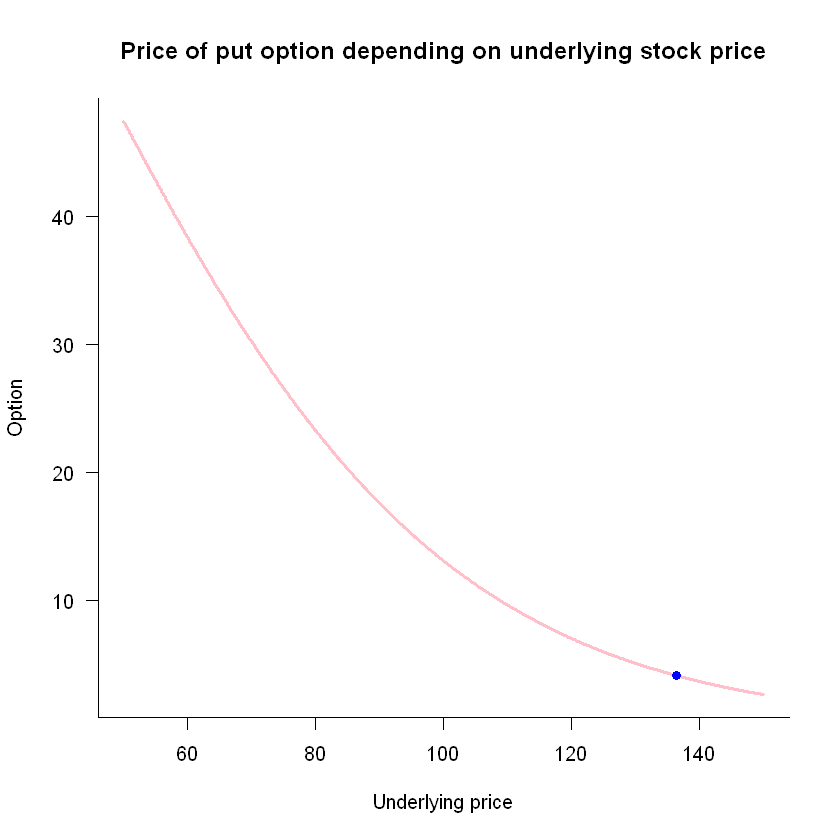We can see the put price is decreasing on the underlying price, and convex.

## Simulate option prices with Monte Carlo¶

A Monte Carlo method is a class of computational algorithm that uses repeated random sampling to obtain numerical results. It is commonly used in quantitative finance to simulate uncertainty and analyze instruments, portfolios or investments.

We are going to do a simple exercise to simulate the profit and loss distribution from holding a stock.

We can use a random number generator to simulate the price of an option:

In :
# Using Monte Carlo

# Simulations
S <- 1e6

# Set seed for replicability
set.seed(420)

# Get 1e6 simulations from a standard normal
ysim <- rnorm(S)

# Plot the random numbers
hist(ysim)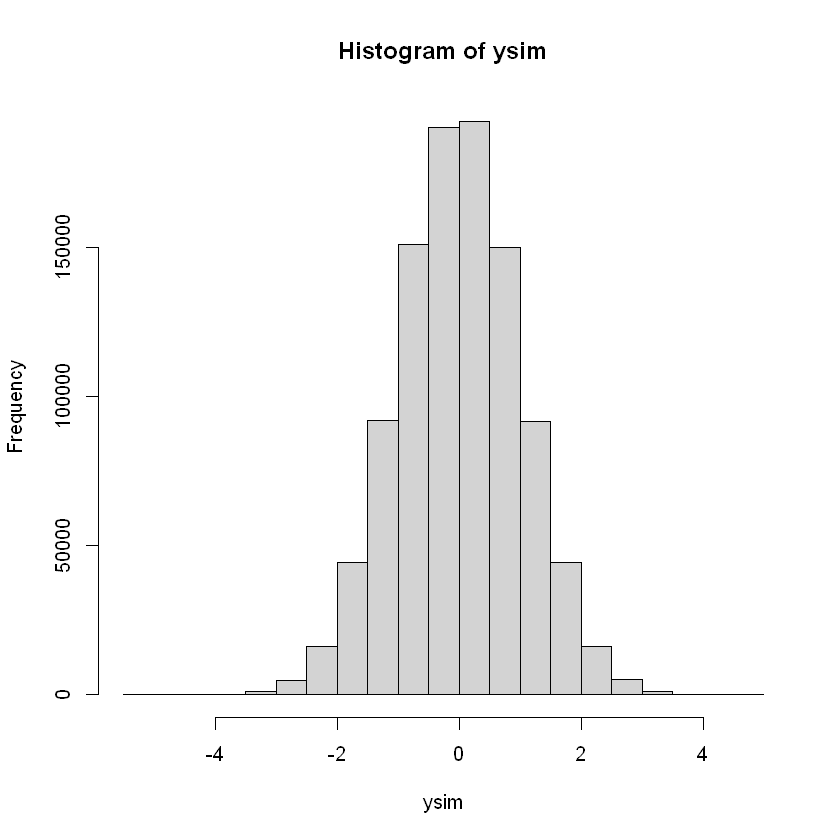Remember we need to apply a log-normal correction in the simulation. To do so, we subtract $\frac{1}{2}\sigma^2$ from the simulated stock returns. By default, rnorm() uses a standard normal, we would also need to scale for the desired variance:

In :
# Centering in the desired mean and changing the variance
ysim <- ysim * sigma * sqrt(Maturity)
ysim <- ysim - 0.5 * sigma^2 * Maturity


We can directly specify this in rnorm() to account for the variance and log-normal correction:

In :
# Specifying mean/sd in rnorm directly
ysim <- rnorm(S, mean = -0.5 * sigma^2 * Maturity, sd = sigma * sqrt(Maturity))

# Histogram
hist(ysim, probability = TRUE)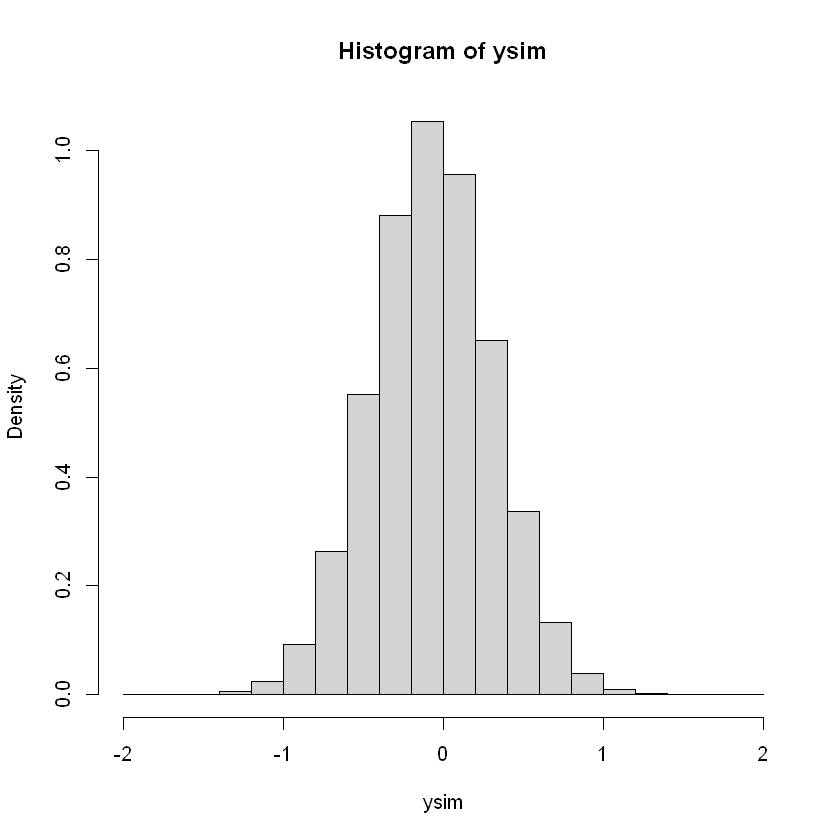Now we can simulate the no-arbitrage future prices of the stock:

In :
# Simulating future prices of stock
Fsim <- P * exp(r * Maturity) * exp(ysim)
hist(Fsim, probability = TRUE)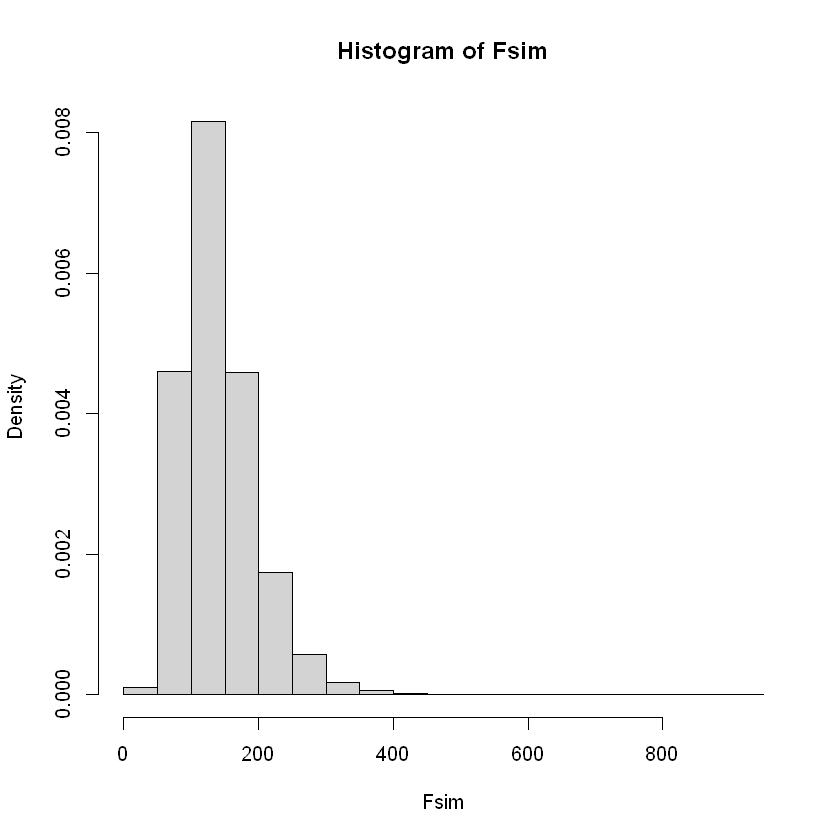To simulate the price of the put option, we subtract the simulated prices of the underlying stock from the strike price, and replace it with 0 if negative, since the option would not be executed:

In :
# Simulated price of stock
Psim <- K - Fsim

# Replacing negatives with zero
Psim[Psim<0] <- 0

# Histogram
hist(Psim, probability = TRUE)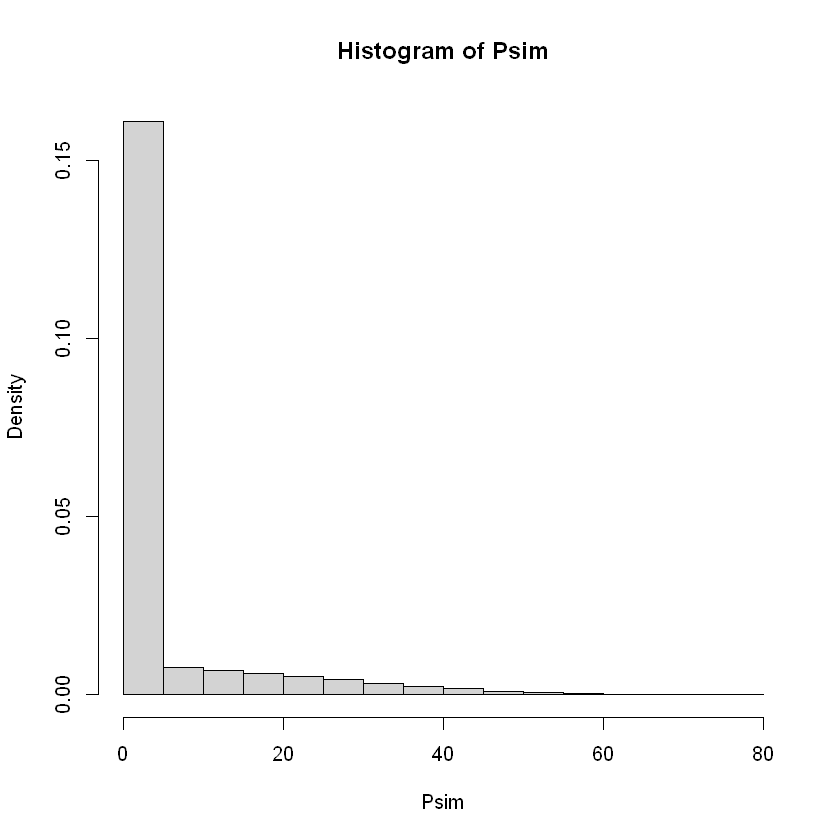The histogram has a peak in 0 due to all the cases when the put option is not executed. Now let's compare the price we obtained analytically using the Black-Scholes formula and the price obtained through Simulation:

In :
# Comparing analytical and simulation prices
Psim <- Psim * exp(-r * Maturity)

cat("Analytic price:", put, "\n",
"Simulation price:", mean(Psim))

Analytic price: 4.116631
Simulation price: 4.172611

## Analytical and simulations method for VaR¶

Let's first find the VaR of the stock using a simulation:

In :
# Simulating arithmetic returns

# Specifying VaR probability
probability <- 0.01

# Sample standard deviation
sigma <- sd(y)

# Number of simulations
S <- 1e3

# Set a seed
seed <- 456
set.seed(seed)

# Draw from a normal distribution, using daily rate and standard deviation
ysim <- rnorm(S, mean = r/365, sd = sigma)

# Simulate prices
Psim <- P * (1 + ysim)

# Sort the simulated profit/loss
q <- sort(Psim - P)

# VaR from quantile
VaR1s <- -q[ceiling(probability*S)]

# Round
round(VaR1s,2)

7.35

We can compare it with the analytical VaR:

In :
# Analytical VaR
VaR1t <- abs(sigma * qnorm(probability) * P)
round(VaR1t,2)

7.47

## Calculating VaR for the option¶

To calculate the VaR for the option we can apply the Black-Scholes formula using the observed price and the simulated prices, and a sigma scaled for the yearly trading days:

In :
# VaR for the option

# Calculate put
put <- bs(K = K, P = P, r = r, sigma = sqrt(sigma^2 * 250), Maturity = Maturity)

# Simulate prices
S <- 1e3
set.seed(444)
ysim <- rnorm(S, mean = r/365, sd = sigma)
Psim <- P * (1 + ysim)

# Use simulated prices in Black-Scholes formula
fsim <- bs(K = K, P = Psim, r = r, sigma = sqrt(sigma^2 *250),
Maturity = Maturity - (1/365))

# Sort the vector
q <- sort(fsim - put)

# Get the value that meets the VaR probability
VaR2 <- -q[ceiling(probability*S)]
round(VaR2,3)

0.905

## Calculating VaR for a portfolio of stock and option (Not covered in class)¶

If we have a portfolio that has both an option and the underlying stock, we can calculate VaR as follows:

In :
# VaR for a portfolio

# Assets
xb <- 3
xo <- 2

# Create portfolio
portfolio_today <- xb*P + xo*put
round(portfolio_today)

417

Use the vector of simulated prices, Psim, to calculate tomorrow's value of the portfolio:

In :
# Calculating tomorrow's value of the portfolio

option_tomorrow <- bs(K = K, P = Psim, r = r, sigma = sqrt(sigma^2 * 250), Maturity = Maturity - (1/365))
portfolio_tomorrow <- xb*Psim + xo*option_tomorrow

In :
# Find the profit-loss
portfolio_loss <- portfolio_tomorrow - portfolio_today

# We can see its distribution
hist(portfolio_loss, main = "P/L distribution")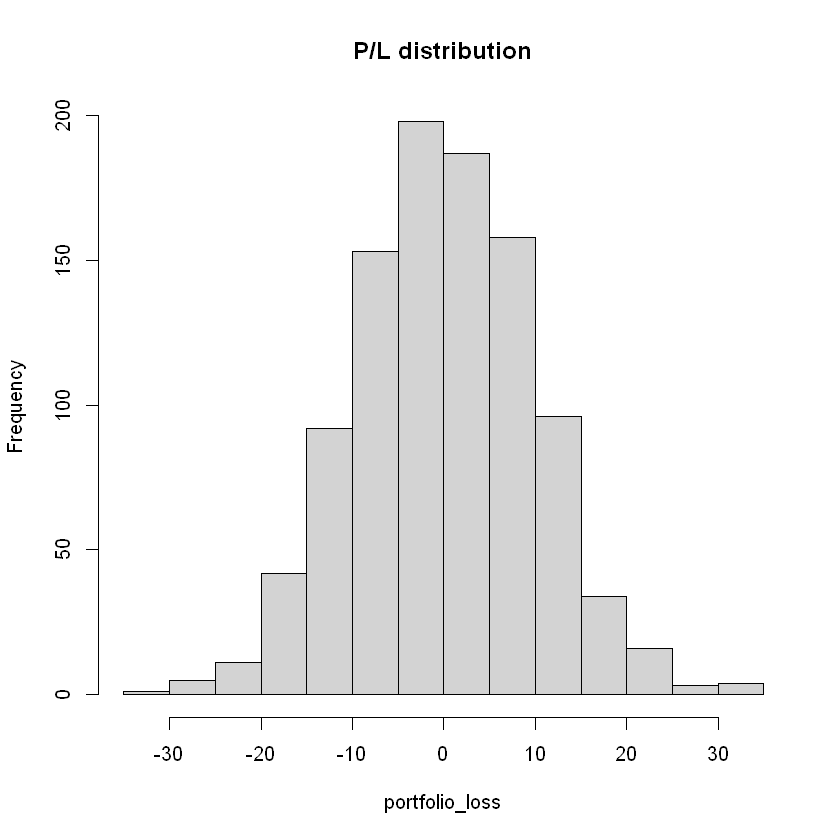In :
# Find VaR
portfolio_loss <- sort(portfolio_loss)
portfolio_VaR <- -portfolio_loss[ceiling(S*probability)]
round(portfolio_VaR,2)

22.73

We can include a new option, with a different strike price, and see how it changes our VaR:

In :
# Introducing a second option
xo2 <- 15
K2 <- 90

# Get the prices using Black-Scholes
option2_today <- bs(K=K2, P=P, r=r, sigma=sqrt(sigma^2 *250), Maturity=Maturity)
option2_tomorrow <- bs(K=K2, P=Psim, r=r, sigma=sqrt(sigma^2 * 250), Maturity=Maturity-(1/365))

# Portfolio values
portfolio_today <- xb*P + xo*put + xo2*option2_today
portfolio_tomorrow <- xb*Psim + xo*option_tomorrow + xo2*option2_tomorrow

# Find the profit-loss
portfolio_loss <- portfolio_tomorrow - portfolio_today

# We can see its distribution
hist(portfolio_loss, main = "P/L distribution")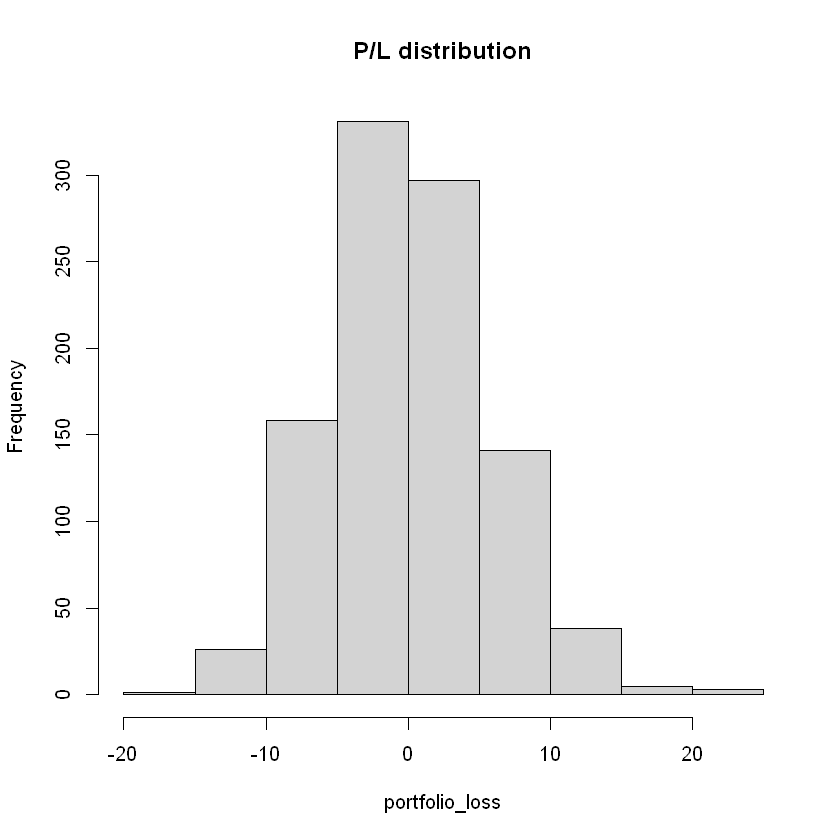In :
# Find VaR
portfolio_loss <- sort(portfolio_loss)
portfolio_VaR <- -portfolio_loss[ceiling(S*probability)]
round(portfolio_VaR,2)

12.05

## Recap¶

In this seminar we have covered:

• Pricing a put option analytically with Black-Scholes
• Simulating option prices with Monte Carlo
• Evaluating convergence to analytical solutions for different simulation sizes
• Stock, option and portfolio VaR calculations

For more discussion on the material covered in this seminar, refer to Chapter 6: Analytical value-at-risk for options and bonds and Chapter 7: Simulation methods for VaR for options and bonds on Financial Risk Forecasting by Jon Danielsson.

Acknowledgements: Thanks to Alvaro Aguirre and Yuyang Lin for creating these notebooks